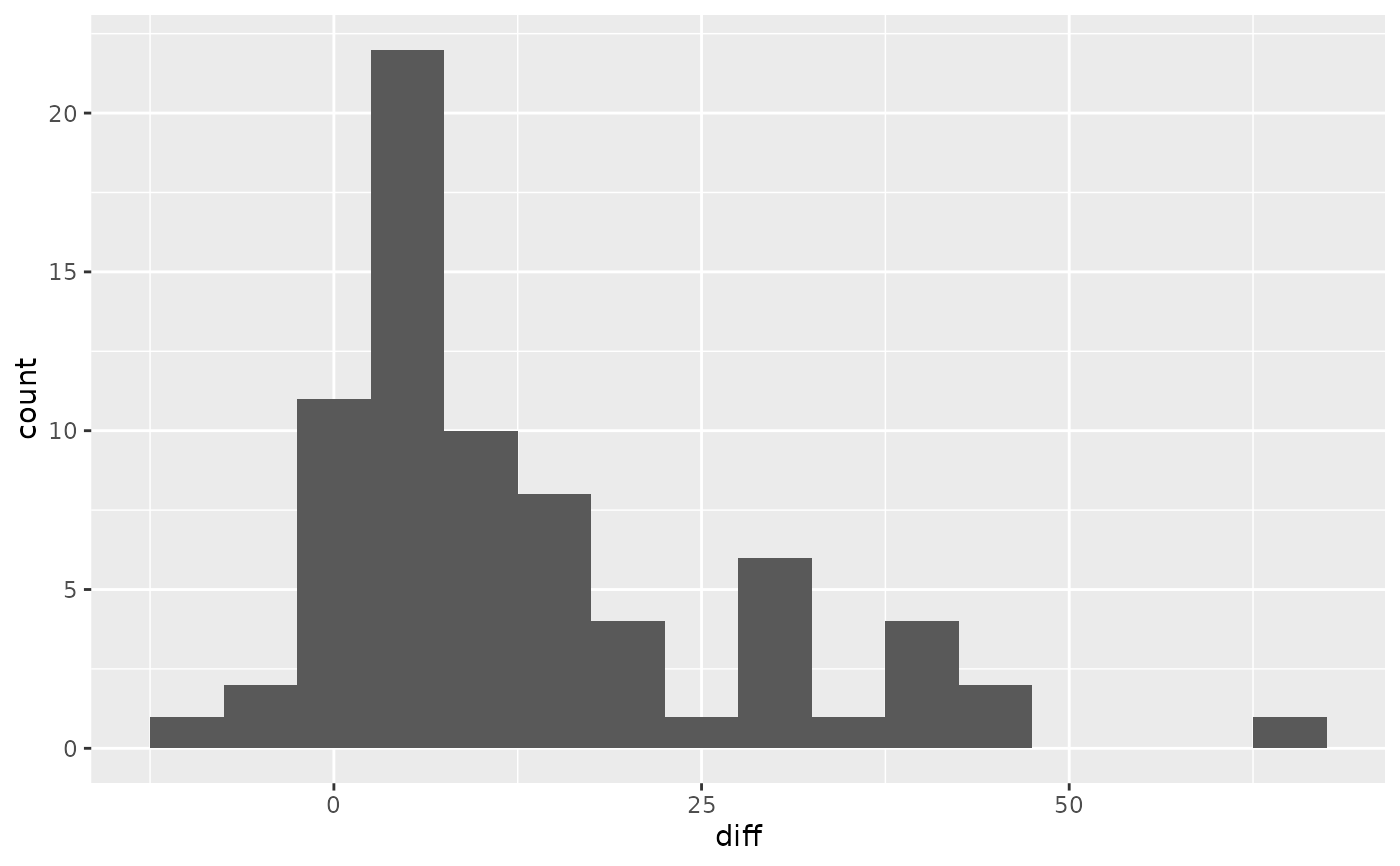A random sample was taken of nearly 10\ textbook for each course was identified, and its new price at the UCLA Bookstore and on Amazon.com were recorded.

## Usage

textbooks

## Format

A data frame with 73 observations on the following 7 variables.

dept_abbr

Course department (abbreviated).

course

Course number.

isbn

Book ISBN.

ucla_new

New price at the UCLA Bookstore.

amaz_new

New price on Amazon.com.

more

Whether additional books were required for the course (Y means "yes, additional books were required").

diff

The UCLA Bookstore price minus the Amazon.com price for each book.

## Source

Collected by David Diez.

## Details

The sample represents only courses where textbooks were listed online through UCLA Bookstore's website. The most expensive textbook was selected based on the UCLA Bookstore price, which may insert bias into the data; for this reason, it may be beneficial to analyze only the data where more is "N".

## Examples


library(ggplot2)

ggplot(textbooks, aes(x = diff)) +
geom_histogram(binwidth = 5)t.test(textbooks$diff) #> #> One Sample t-test #> #> data: textbooks$diff
#> t = 7.6488, df = 72, p-value = 0.00000000006928
#> alternative hypothesis: true mean is not equal to 0
#> 95 percent confidence interval:
#>   9.435636 16.087652
#> sample estimates:
#> mean of x
#>  12.76164
#>